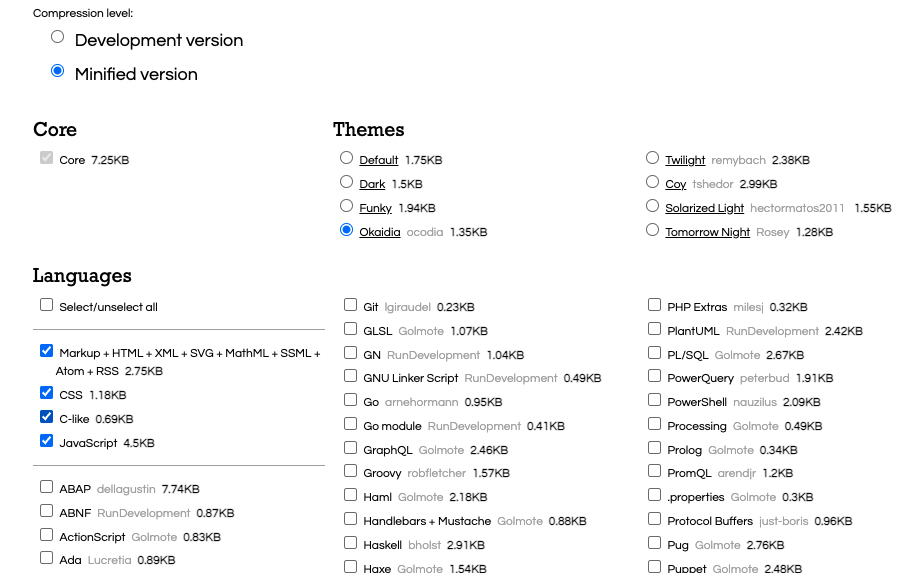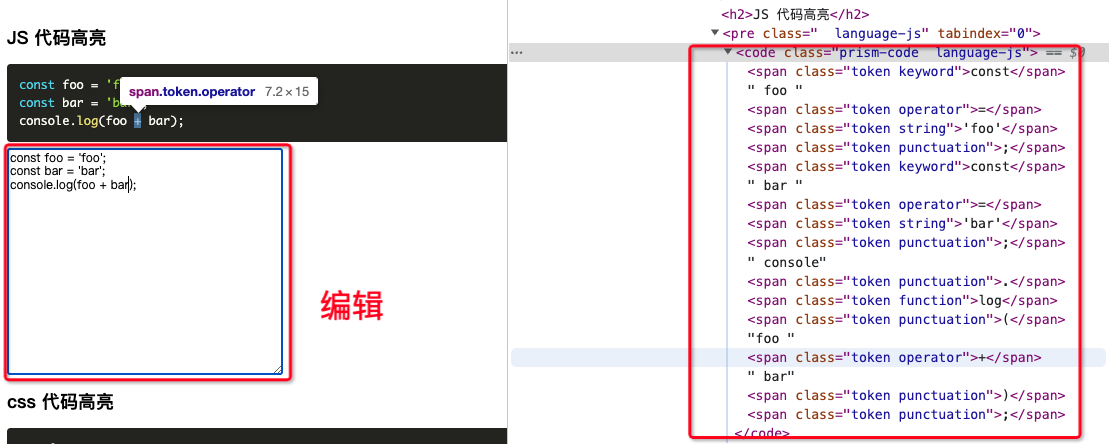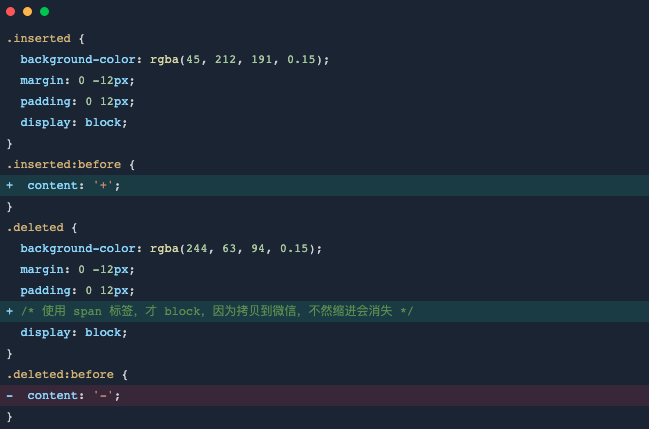Published on

# 使用 Prism.js 对代码进行语法高亮

Authors
•作者
狂奔滴小马

## Prism.js 的使用``````<!DOCTYPE html>
<html>
<meta charset="UTF-8" />
<meta name="viewport" content="width=device-width, initial-scale=1.0" />
<body>
<pre><code class="language-css">p { color: red }</code></pre>
<script>
window.Prism = window.Prism || {}
window.Prism.manual = false
</script>
<script src="prism.js"></script>
</body>
</html>
``````

``````<script src="prism.js" data-manual></script>
``````

``````<script>
window.Prism = window.Prism || {}
window.Prism.manual = true
</script>
<script src="prism.js"></script>
``````

## 在 react 中使用

``````npm install --save prismjs
# 或者
``````

``````npm install --save-dev babel-plugin-prismjs
# 或者
``````

``````{
"plugins": [
[
"prismjs",
{
"languages": ["javascript", "css", "html"],
"plugins": ["line-numbers", "show-language"],
"theme": "okaidia",
"css": true
}
]
]
}
``````

## 实现 react 组件

``````import React, { useRef, useEffect } from 'react'

function PrismCode({ code, language, plugins = [] }) {
const ref = useRef(null)
useEffect(() => {
if (ref && ref.current) {
Prism.highlightElement(ref.current)
}
}, [code])

return (
<pre className={plugins.join(' ')}>
<code ref={ref} className={`prism-code language-\${language}`}>
{code}
</code>
</pre>
)
}
``````

``````import React from 'react'
import { PrismCode } from './component'
const code = `
const foo = 'foo';
const bar = 'bar';
console.log(foo + bar);
`
const Example = () => <PrismCode code={code} language="js" plugins={['line-numbers']} />
``````

## 高级技巧## prism-react-renderer

``````# npm
npm install --save prism-react-renderer
# yarn
``````

``````import React from "react";
import { render } from "react-dom";
import Highlight, { defaultProps } from "prism-react-renderer";

const exampleCode = `
(function someDemo() {
var test = "Hello World!";
console.log(test);
})();

return () => <App />;
`;

render((
<Highlight {...defaultProps} code={exampleCode} language="jsx">
{({ className, style, tokens, getLineProps, getTokenProps }) => (
<pre className={className} style={style}>
{tokens.map((line, i) => (
<div {...getLineProps({ line, key: i })}>
{line.map((token, key) => (
<span {...getTokenProps({ token, key })} />
))}
</div>
))}
</pre>
)}
</Highlight>,
document.getElementById('root')
);
``````

## 代码行高亮

``````const isDiff = language.startsWith('diff-')

let highlightStyle = []

let code = children
if (isDiff) {
code = []
language = language.substr(5)
highlightStyle = children.split('\n').map((line) => {
if (line.startsWith('+')) {
code.push(line.substr(1))
return 'inserted'
}
if (line.startsWith('-')) {
code.push(line.substr(1))
return 'deleted'
}
code.push(line)
})
code = code.join('\n')
}
``````

## 使用